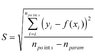# Linear function errors

• TheDestroyer

#### TheDestroyer

Hi,

When I'm doing an experiment,

If the result of the experiment was some points, which I interpolate to create the linear function:

y=(a/b^2) x+c

while y is a function of x: y=f(x)

If the standard error in y was (S), How can I calculate the error in b?

Thank you

Are you using "least square fit" ?

No, I'm using the statistical way, you can see its law in the image I've attached,

(((n_points is the quantity of the used points, y_i is the y-coordinate of the point i due to experiment, f(x_i) is the y-coordinate of the point i due to the interpolated function, n_param is the repeat of every point which equals always one as I think in my experiment because every point is measured once)))

I'm using program named "curve expert 1.3" to interpolate the linear function, you can download it for free and check the errors details, i was not able to upload the detailed help file because it's big

Is this information enough?

#### Attachments

•StandardError.jpg
18.3 KB · Views: 345
Last edited:
Well these programs are very often doing least square fit.. but the calculate it very fast hehe.

Least square fit is a statistical method.

Scince you are using a program that i don't use, i can't help you. sorry.